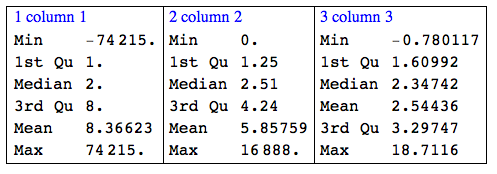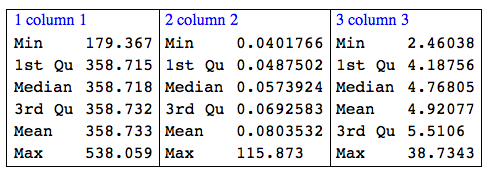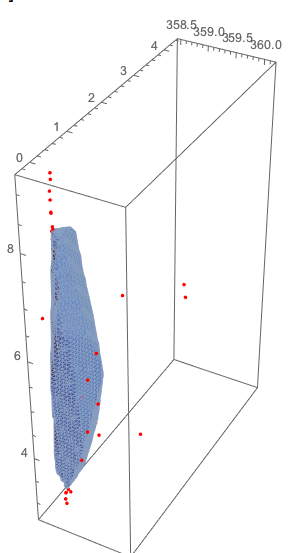# Finding outliers in 2D and 3D numerical data

## Introduction

This blog post describes a method of finding outliers in 2D and 3D data using Quantile Regression Envelopes discussed in previous blog posts: “Directional quantile envelopes”, “Directional quantile envelopes in 3D”.

## Data

In order to provide a good example of the method application it would be better to use “real life” data. I found this one: “UCI Online Retail Data Set”. That dataset has columns for online purchase transactions (quantity and price).

``````(*data=Import["http://archive.ics.uci.edu/ml/machine-learning-databases/00352/Online%20Retail.xlsx","XLSX"];*)
``````

so I downloaded the XLSX file and saved it into a CSV file, then imported it:

``````data = Import["~/Datasets/UCI Online Retail Data Set/Online Retail.csv",
"IgnoreEmptyLines" -> True, "HeaderLines" -> 0];
columnNames = data[];
data = Rest[data];
``````

Here are the dimensions of the dataset (seems “realistically” large):

``````Dimensions[data]
(* {65499, 8} *)
``````

Here is a summary of the quantative columns:

``````Grid[{RecordsSummary[N@data[[All, {4, 6}]], columnNames[[{4, 6}]]]}, Dividers -> All]
``````Since we have only two quantative columns in the data let us add a third one, and make it have 3 outliers.

``````dvec = RandomReal[SkewNormalDistribution[1, 2, 4], Dimensions[data][]];
Block[{inds = RandomSample[Range[Length[dvec]], 3]},
dvec[[inds]] = 10*dvec[[inds]]];
testData = MapThread[Append, {N@data[[All, {4, 6}]], dvec}];
``````

Here is the summary:

``````Grid[{RecordsSummary[testData]}, Dividers -> All]
``````### Standardizing

It is a good idea to standardize the data. This is not necessary for the outlier finding procedure used below, but it makes the data more convenient for visualization or other exploration.

``sTestData = Transpose[Standardize /@ Transpose[N@testData]];``

Because of the outliers plotting the data might produce uninformative plots. We can use logarithms of the point coordinates and for that we have to shift the standardized data to be positive. This is done with this command:

``````Block[{offset = -2 (Min /@ Transpose[sTestData])},
sTestData = Map[# + offset &, sTestData]];
``````

Let use show the standardized data summary and visualize:

``Grid[{RecordsSummary[sTestData]}, Dividers -> All]````````opts = {PlotRange -> All, ImageSize -> Medium,
PlotTheme -> "Detailed"}; Grid[{{ListPointPlot3D[sTestData, opts],
ListPointPlot3D[Log10@sTestData, opts]}}]
``````## Using Quantile Regression envelopes to find outliers

We are going to find the outliers by computing envelopes around the dataset points that contain almost all points (e.g. 99.7% of them).

The finding of directional quantile envelopes in 2D and 3D is explained in these blog posts:

I am a big fan of Quantile Regression (QR) and I have implemented a collection of functions and applications of QR. See these blog posts.

This command imports the package QuantileRegression.m :

``````Import["https://raw.githubusercontent.com/antononcube/MathematicaForPrediction/master/QuantileRegression.m"]
``````

Here is an example of an envelope found using directional quantiles over a small sample of the points:

``````Block[{testData = RandomSample[sTestData, 2000], qreg},
qreg = QuantileEnvelopeRegion[testData, 0.997, 10];
Show[{Graphics3D[{Red, Point[testData]}, Axes -> True],
BoundaryDiscretizeRegion[qreg]}]
]
``````The outlier points (in red) are outside of the envelope.

The example (and plot) above are just for illustration purposes. We calculate a quantile evelope region using all points.

``````Block[{testData = sTestData},
qreg = QuantileEnvelopeRegion[testData, 0.9997, 10];
]
``````

(The function `QuantileEnvelopeRegion` works also for 2D. For 2D nothing else changes in the steps below except using 2D graphics.)

This command makes a function to test does a point belong to the found envelope region or not:

``````rmFunc = RegionMember[qreg];
``````

This calculates the membership predicates for all points:

``````AbsoluteTiming[
pred = rmFunc /@ sTestData;
]

(* {15.6485, Null} *)
``````

And we can see the membership breakdown:

``````Tally[pred]
(* {{True, 65402}, {False, 97}} *)
``````

and visualize it (using `Pick` and taking logarithms):

``````Graphics3D[{Gray, Point[Log10@Pick[sTestData, pred]], Red,
Point[Log10@Pick[sTestData, Not /@ pred]]}, Axes -> True]
``````The plot above contains both top and bottom outliers (in red). If we are intereseted only in the top outliers we can find these thresholds:

``````topThresholds = Quantile[#, 0.95] & /@ Transpose[testData]
(* {25, 10.95, 4.89433} *)
``````

and use them to select the top outliers:

``````Select[Pick[testData, Not /@ pred],
Total[Thread[# > topThresholds] /. {True -> 1, False -> 0}] > 1 &]

(* {{1, 836.14, 6.48564}, {1, 16.13, 8.71455}, {5, 25.49, 8.47351}, {-1, 1126, 5.25211}, {1000, 0, 5.4212}, {1, 15.79, 9.14674}, {-1, 544.4, 6.86266}} *)
``````

Note that the quantile regression envelope and the membership predicates were computed over the standardized data, and the predicates were used to retrieve the outliers of the original data.